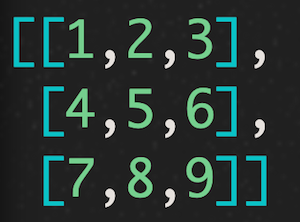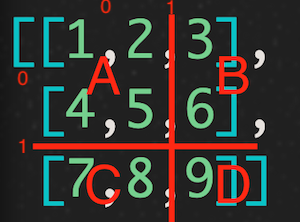# How to divide a two-dimensional array into four with ruby

Due to various reasons, I created a method that is profitable for everyone. I think the letters are refreshing, so I wrote a picture!

For example, suppose you have an arbitrary two-dimensional array like this.I want to specify arbitrary coordinates as shown in (1,1) and divide it into four.The code is here.

``````def divide_2d_array(array, x, y)
temp1 = []
temp2 = []
array.each do |a|
temp1 << a[0..y]
temp2 << a[(y+1)..-1]
end
a,c = temp1[0..x], temp1[(x+1)..-1]
b,d = temp2[0..x], temp2[(x+1)..-1]
return a,b,c,d
end

#I will try to run
arr = [[1,2,3],
[4,5,6],
[7,8,9]]

p divide_2d_array(arr, 1, 1)
# =>[[[1, 2], [4, 5]], [, ], [[7, 8]], []]
``````

It's done! !! !! Please let me know if there is a better way!